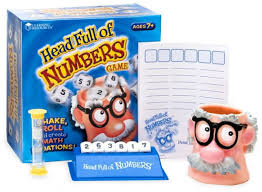• 1899

Kids will have math on their minds with this game for any number of players! From one child to the whole family, everyone will love the fast-paced fun.

Place the three standard dice and three custom dice (with numbers 0, 1, 2, 7, 8 and 9) into the fun dice shaker then roll them out and place them into the dice tray. Set the sand timer and have the score pad ready to record the number of unique, correct equations each player makes using the numbers rolled. Equations can use simple addition or subtraction or include multiplication and division for a more challenging game.

Guide included.

Ages 7-12
2-6 Players or Groups
10 Minutes to Learn
10-30 Minutes to Play

Game Play:
1. Place the six dice into the shaker.

2. Have one player roll the dice out of the head.

3. Once the dice are rolled, place the six dice in the tray, with the numbers rolled facing up. These are the numbers that will be used during gameplay.

4. Each player should then write the six numbers at the top of the scorecard in the spaces provided.

5. Start the timer. All players write as many equations as they can during that time.

6. Many forms of equations can be made: +, -, x, /, =, <, and > are all possibilities. The trick is to find a combination that works using only the numbers rolled.

For example, the numbers rolled were 7, 2, 2, 3,
6, 4. The equations below are possible solutions that could be made.

4 + 2 = 6 6 x 4 = 24 2+4=6 6÷3=2 2=2 6÷2=3 6–4=2 7+2=6+3

24 ÷ 6 = 4 26 – 24 = 2 6>4 4<6

7. A number can be used only once in an equation if only one of that number was rolled. However, the answer may repeat a number that was used to create the equation.

(For example, with the numbers rolled in the example above you can create 24 ÷ 6 = 4 even though only one 4 was rolled.)

*Exception: You may not repeat a number when creating an equal-to equation (ex. 3 = 3)

if there is only one of that number rolled.

8. Reverse equations are also allowed. (For example, 4 + 2 = 6 and 2 + 4 = 6)

9. Once the time runs out, the players say their equations aloud.

Scoring

2. Any correct equation that is unique from the other players’ equations earns two points.

3. The player who had the most correct equations total earns an additional three points. (If there is a tie, no
extra points are awarded.)

4. Gameplay continues until one player reaches 50 points or another specified total.

Ages 7-12
2-6 Players or Groups
10 Minutes to Learn

We Also Recommend